# rectangularArray

Create rectangular antenna array

## Description

The `rectangularArray` class creates a rectangular antenna array in the X-Y plane. By default, the rectangular array is a four-element dipole array in a 2 x 2 rectangular lattice. The dipoles are center-fed. Each dipole resonates at 70 MHz when isolated.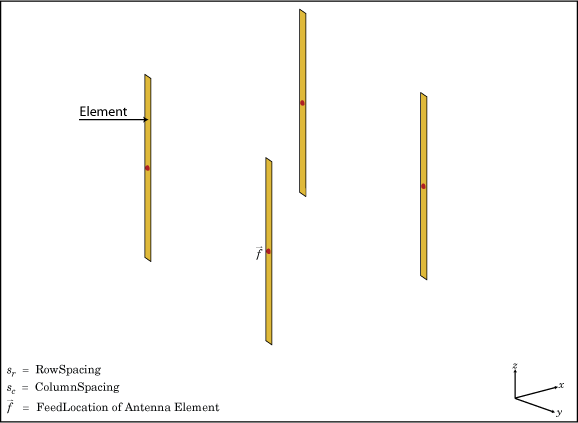## Creation

### Syntax

``array = rectangularArray``
``array = rectangularArray(Name,Value)``

### Description

example

``` `array = rectangularArray` creates a rectangular antenna array in the X-Y plane. ```

example

``` `array = rectangularArray(Name,Value)` creates a rectangular antenna array, with additional properties specified by one or more name-value pair arguments. `Name` is the property name and `Value` is the corresponding value. You can specify several name-value pair arguments in any order as `Name1`, `Value1`,``` ...```, `NameN`, `ValueN`. Properties not specified retain default values.```

### Output Arguments

expand all

Rectangular array, returned as an `rectangularArray` object.

## Properties

expand all

Antenna elements or linear arrays, specified as an antenna or array object.

Example: `'Element',monopole`

Number of antenna elements in row and column of array, specified as a two-element vector.

Example: `'Size',[4 4]`

Row spacing between two antenna elements, specified as a scalar or vector in meters. By default, the antenna elements are spaced `2m` apart.

Example: `'RowSpacing'`,[5 6]

Data Types: `double`

Column spacing between two antenna elements, specified as a scalar or vector in meters. By default, the antenna elements are spaced `2m` apart.

Example: `'ColumnSpacing',[3 4]`

Data Types: `double`

Antenna elements spatial arrangement, specified as a text input.

Example: `'Lattice'`,`"Triangular"`

Data Types: `char` | `string`

Excitation amplitude of antenna elements, specified as a scalar or vector. Set the property value to `0 ` to model dead elements.

Example: `'AmplitudeTaper',3`

Data Types: `double`

Phase shift for antenna elements, specified as a scalar or vector in degrees.

Example: `'PhaseShift',[3 3 0 0]`

Data Types: `double`

Tilt angle of the array specified as a scalar or vector with each element unit in degrees. For more information, see Rotate Antennas and Arrays.

Example: `Tilt=90`,

Example: `Tilt=[90 90]`,`TiltAxis=[0 1 0;0 1 1]` tilts the array at 90 degrees about the two axes, defined by vectors.

Data Types: `double`

Tilt axis of the array, specified as:

• Three-element vectors of Cartesian coordinates in meters. In this case, each vector starts at the origin and lies along the specified points on the X-, Y-, and Z-axes.

• Two points in space, each specified as three-element vectors of Cartesian coordinates. In this case, the array rotates around the line joining the two points in space.

• A string input describing simple rotations around one of the principal axes, 'X', 'Y', or 'Z'.

Example: `TiltAxis=[0 1 0]`

Example: `TiltAxis=[0 0 0;0 1 0]`

Example: `TiltAxis='Z'`

Data Types: `double`

## Object Functions

 `show` Display antenna, array structures or shapes `info` Display information about antenna or array `beamwidth` Beamwidth of antenna `charge` Charge distribution on antenna or array surface `correlation` Correlation coefficient between two antennas in array `current` Current distribution on antenna or array surface `design` Design prototype antenna or arrays for resonance around specified frequency `efficiency` Radiation efficiency of antenna `EHfields` Electric and magnetic fields of antennas; Embedded electric and magnetic fields of antenna element in arrays `impedance` Input impedance of antenna; scan impedance of array `layout` Display array or PCB stack layout `mesh` Mesh properties of metal, dielectric antenna, or array structure `meshconfig` Change mesh mode of antenna structure `optimize` Optimize antenna or array using SADEA optimizer `pattern` Radiation pattern and phase of antenna or array; Embedded pattern of antenna element in array `patternAzimuth` Azimuth pattern of antenna or array `patternElevation` Elevation pattern of antenna or array `rcs` Calculate and plot radar cross section (RCS) of platform, antenna, or array `returnLoss` Return loss of antenna; scan return loss of array `sparameters` Calculate S-parameter for antenna and antenna array objects

## Examples

collapse all

Create and plot the layout of a rectangular array of four dipoles.

```ra = rectangularArray; ra.Size = [2 2]; layout(ra);```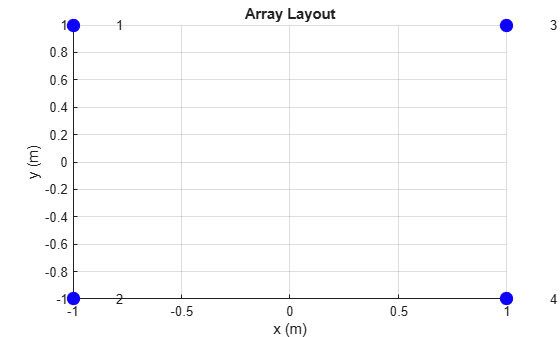Calculate the scan impedance of a 2x2 rectangular array of dipoles at 70 MHz.

```h = rectangularArray('Size',[2 2]); Z = impedance(h,70e6)```
```Z = 1×4 complex 26.7086 -56.9363i 26.6918 -56.9498i 26.7086 -56.9363i 26.6918 -56.9498i ```

Create a rectangular array of monopoles.

```m1 = monopole; mra = rectangularArray('Element',m1); show(mra);```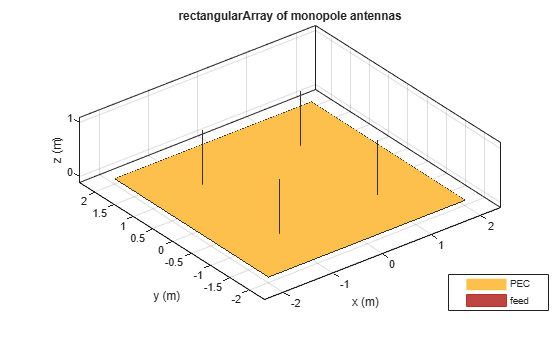Create an array of discones with element spacing of 3 m.

```la = linearArray('Element',discone); la.ElementSpacing = 3; show(la)```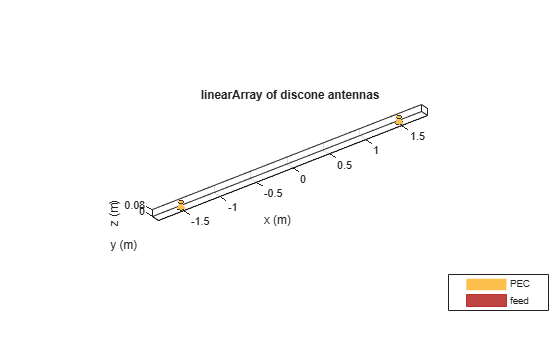Create a rectangular of the linear array.

`ra = rectangularArray("Element",la)`
```ra = rectangularArray with properties: Element: [1x1 linearArray] Size: [2 2] RowSpacing: 2 ColumnSpacing: 2 Lattice: 'Rectangular' AmplitudeTaper: 1 PhaseShift: 0 Tilt: 0 TiltAxis: [1 0 0] ```
`show(ra)`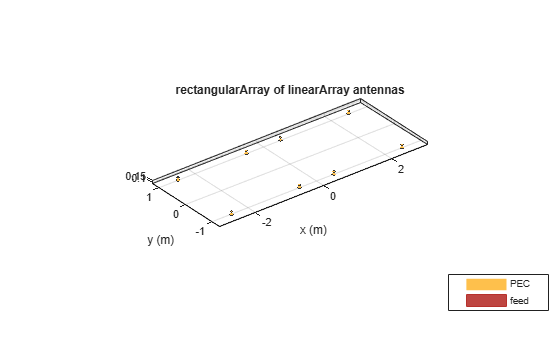Balanis, C.A. Antenna Theory. Analysis and Design, 3rd Ed. New York: Wiley, 2005.